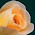## Sunday, May 25, 2014

### PCalc and HP 50g: Mohr's CirclePCalc and HP 50g: Mohr's Circle

Given shear stresses in the x and y directions (σx and σy) and normal stress (τ), find the radius, center, and viewing angle of Mohr's Circle.

I take τ to represent both τxy and τyx (both are assumed to be equal).

R = √( (σx - σy)/2)^2 - τ^2 )

Center (S, 0):
S = (σx + σy)/2

Viewing Angle:
θ = atan( 2τ /(σx - σy)) / 2

Sources:

HP 35S Program by Jason Charalambides, Avant Garde Engineering. HP 35S program written in 2012.

http://www.avant-garde-engineering.com/HP35s_Programs/Mohr's%20Circle.pdf

Wikipedia Article on Mohr's Circle, retrieved 5/23/2014:

http://en.wikipedia.org/wiki/Mohr's_circle

PCalc:

Before running, store the following values:
σx into M1
σy into M2
τ into M3

Program: Mohr's Circle - Radius
Decimal Mode
Set X To M1
Subtract M2 From X
Divide X By 2
X To Power of 2
Set R0 To M3
R0 To Power of 2
Add R0 To X
X To Power of 0.5

Program: Mohr's Circle - Center (X,0)
Decimal Mode
Set X To M1
Add M2 To X
Divide X By 2

Program: Mohr's Circle - View Angle
Decimal Mode
Set X To M3
Multiply X By 2
Set R0 To M1
Subtract M2 From M0
Divide X By R0
Inverse Tangent X
Divide X By 2

HP 50g: MOHR

σ: ALPHA, Right-Shift, S
τ: ALPHA, Right-Shift, U
SQ: x^2

<< "Shear Stress σx" PROMPT
"Shear Stress σy" PROMPT
"Normal Stress τ" PROMPT
→ X Y T
<< X Y - 2 / SQ T SQ + √
X Y + 2 / (0,0) +
"Center" →TAG
T 2 * X Y - / ATAN 2 /
"View Angle" →TAG >> >>

Example:
σx = 100
σy = -220
τ = 80

Results:
R ≈ 178.88544
S = -60 (center (-60,0))
θ ≈ 13.28253° ≈ .23183 radians

- Eddie -

#### 1 comment:

1.I think you have your naming conventions reversed. Sigma x and sigma y are normal stresses and tau is the shear stress

### Retro Review: Radio Shack EC-2024

Retro Review:  Radio Shack EC-2024 Quick Facts Company:  Radio Shack Years in Production: Around 1994 Original Price: \$24.99 Bat...